# Solving Linear Inequalities Word Problems

• Last Updated : 28 May, 2021

We are well versed with equations in multiple variables. Linear Equations represent a point in a single dimension, a line in a two-dimensional, and a plane in a three-dimensional world. Solutions to linear inequalities represent a region of the Cartesian plane. It becomes essential for us to know how to translate real-life problems into linear inequalities.

### Linear Inequalities

Before defining the linear inequalities formally, let’s see them through a real-life situation and observe why their need arises in the first place. Let’s say Albert went to buy some novels for himself at the book fair. He has a total of Rs 200 with him. The book fair has a special sale policy which offers any book at Rs 70. Now he knows that he may not be able to spend the full amount on the books. Let’s say x is the number of books he bought. This situation can be represented mathematically by the following equation,

70x < 200

Since he can’t spend all the amount on books, and also the amount spent by him will always be less than Rs 200. The present situation can only be represented by the equation given above. Now let’s study the linear inequalities with a formal description,

Two real numbers or two algebraic expressions which are related by symbols such as ‘>’, ‘<‘, ‘≥’ and’≤’ form the inequalities. Linear inequalities are formed by linear equations which are connected with these symbols. These inequalities can be further classified into two parts:

1. Strict Inequalities: The inequalities with the symbols such as ‘>’ or ‘<‘.
2. Slack Inequalities: The inequalities with the symbols such as ‘≥’ or ‘≤’.

Rules of Solving Linear Inequalities:

There are certain rules which we should keep in mind while solving linear inequalities.

1. Equal numbers can be added or subtracted from both sides of the inequality without affecting its sign.
2. Both sides of Inequality can be divided or multiplied by any positive number but when they are multiplied or divided by a negative number, the sign of the linear inequality is reversed.

Now with this brief introduction to linear inequalities, let’s see some word problems on this concept.

### Sample Problems

Question 1: Considering the problem given in the beginning. Albert went to buy some novels for himself at the book fair. He has a total of Rs 200 with him. The book fair has a special sale policy which offers any book at Rs 70. Now he knows that he may not be able to spend the full amount on the books. Let’s say x is the number of books he bought. Represent this situation mathematically and graphically.

Solution:

We know that Albert cannot buy books for all the money he has. So, let’s say the number of books he buys is “x”. Then,

70x < 200

⇒ x <To plot the graph of this inequality, put x = 0.

0 <Thus, x = 0 satisfies the inequality. So, the graph for the following inequality will look like,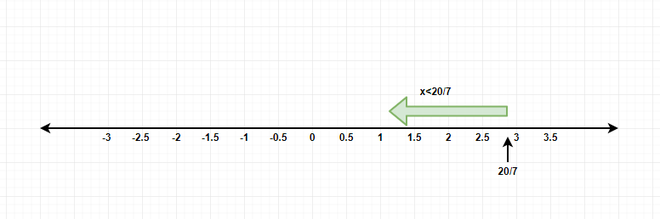Question 2: Consider the performance of the strikers of the football club Real Madrid in the last 3 matches. Ronaldo and Benzema together scored less than 9 goals in the last three matches. It is also known that Ronaldo scored three more goals than Benzema. What can be the possible number of goals Ronaldo scored?

Solution:

Let’s say the number of goals scored by Benzema and Ronaldo are y and x respectively.

x = y + 3 …..(1)

x + y < 9  …..(2)

Substituting the value of x from equation (1) in equation (2).

y + 3 + y < 9

⇒2y  < 6

⇒y < 3

Possible values of y: 0,1,2

Possible values of x: 3,4,5

Question 3: A classroom can fit at least 9 tables with an area of a one-meter square. We know that the perimeter of the classroom is 12m. Find the bounds on the length and breadth of the classroom.

Solution:

It can fit 9 tables, that means the area of the classroom is atleast 9m2. Let’s say the length of the classroom is x and breadth is y meters.

2(x + y) = 12 {Perimeter of the classroom}

⇒ x + y = 6

Area of the rectangle is given by,

xy > 9

⇒x(6 – x) > 9

⇒6x – x2 > 9

⇒ 0 > x2 – 6x + 9

⇒ 0 > (x – 3)2

⇒ 0 > x – 3

⇒ x < 3

Thus, length of the classroom must be less than 3 m.

So, then the breadth of the classroom will be greater than 3 m.

Question 4: Formulate the linear inequality for the following situation and plot its graph.

Let’s say Aman and Akhil went to a stationery shop. Aman bought 3 notebooks and Akhil bought 4 books. Let’s say cost of each notebook was “x” and each book was “y”. The total expenditure was less than Rs 500.

Solution:

Cost of each notebook was “x” and for each book, it was “y”. Then the inequality can be described as,

3x + 4y < 500

Putting (x,y) → (0,0)

3(0) + 4(0) < 500

Origin satisfies the inequality. Thus, the graph of its solutions will look like, x.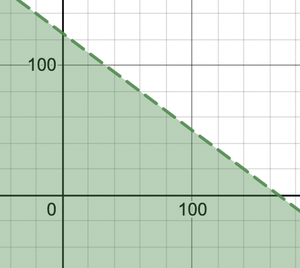Question 5: Formulate the linear inequality for the following situation and plot its graph.

A music store sells its guitars at five times their cost price. Find the shopkeeper’s minimum cost price if his profit is more than Rs 3000.

Solution:

Let’s say the selling price of the guitar is y, and the cost price is x.

y – x > 3000 ….(1)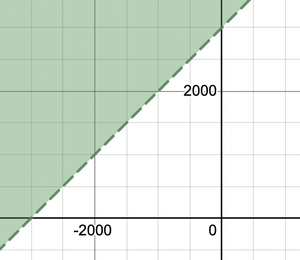It is also given that,

y = 5x ….(2)

Substituting the value of y from equation (2) to equation (1).

5x – x > 3000

⇒ 4x > 3000

⇒  x >⇒ x> 750

Thus, the cost price must be greater than Rs 750.

Question 6: The length of the rectangle is 4 times its breadth. The perimeter of the rectangle is less than 20. Formulate a linear inequality in two variables for the given situation, plot its graph and calculate the bounds for both length and breadth.

Solution:

Let’s say the length is “x” and breadth is “y”.

Perimeter = 2(x + y) < 20 ….(1)

⇒ x + y < 10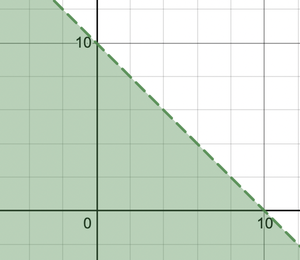Given : x = 4y

Substituting the value of x in equation (1).

x + y < 10

⇒ 5y < 10

⇒ y < 2

So, x < 8 and y < 2.

Question 7: Rahul and Rinkesh play in the same football team. In the previous game, Rahul scored 2 more goals than Rinkesh, but together they scored less than 8 goals. Solve the linear inequality and plot this on a graph.

Solution:

The equations obtained from the given information in the question,

Suppose Rahul scored x Number of goals and Rinkesh scored y number of goals,

Equations obtained will be, x = y+2 ⇢ (1)

x+y< 8 ⇢ (2)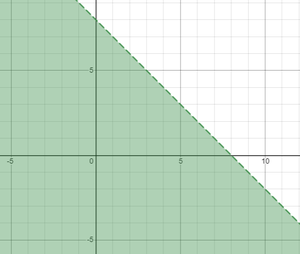Solving both the equations,

y+2 + y < 8

2y < 6

y< 3

Putting this value in equation (2),

x< 5

Question 8: In a class of 100 students, there are more girls than boys, Can it be concluded that how many girls would be there?

Solution:

Let’s suppose that B is denoted for boys and G is denoted for girls.

Now, since Girls present in the class are more than boys, it can be written in equation form as,

G > B

The total number of students present in class is 100 (given),

It can be written as, G+ B= 100

B = 100- G

Substitute G> B in the equation formed,

G> 100 – G

2G > 100

G> 50

Hence, it is fixed that the number of girls has to be more than 50 in class, it can be 60, 65, etc. Basically any number greater than 50 and less than 100.

Question 9: In the previous question, is it possible for the number of girls to be exactly 50 or exactly 100? If No, then why?

No, It is not possible for the Number of girls to be exactly 50 since while solving, it was obtained that, G> 50

In any case if G= 50 is a possibility, from equation G+ B= 100, B = 50 will be obtained.

This simply means that the number of boys is equal to the number of girls which contradicts to what is given in the question.

No, it is not possible for G to be exactly 100 as well, as this proves that there are 0 boys in the class.

Question 10: Solve the linear inequality and plot the graph for the same,

7x+ 8y < 30

x= y/2

Solution:

The linear inequality is given as, 7x+ 8y< 30

At x= 0, y= 30/8= 3.75

At y= 0, x= 30/7= 4.28

These values are the intercepts.

The graph for the above shall look like,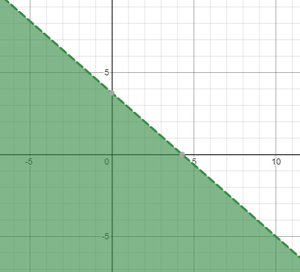Putting x= y/2, that is, y= 2x in the linear inequality,

7x + 16x < 30

x = 1.304

y = 2.609

My Personal Notes arrow_drop_up